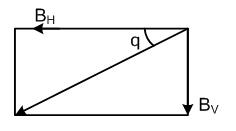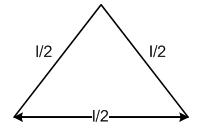×#### Thank you for registering.

One of our academic counsellors will contact you within 1 working day.

Click to Chat

1800-1023-196

+91-120-4616500

CART 0

• 0

MY CART (5)

Use Coupon: CART20 and get 20% off on all online Study Material

ITEM
DETAILS
MRP
DISCOUNT
FINAL PRICE
Total Price: Rs.

There are no items in this cart.
Continue Shopping• Complete Physics Course - Class 11
• OFFERED PRICE: Rs. 2,968
• View Details

```Assignment and Solutions2 for Test

1.         In the above problem the intensity of magnetisation will be (in A/m)-

(A)       7.9×102          (B)       7.9×102          (C)       7.9×102          (D)       7.9×105

Solution:        (D) I – XH = 158.2 × 5000 = 7.9 × 106 Amp

2.         At any place on earth, the horizontal component of earth's magnetic field is √3 times the vertical component. The angle of dip at that place will be-

(A)       60o                  (B)       45o                  (C)       90o                  (D)       30o

Solution:        (D) tan Φ = Bv/BH = Bv / √3 Bv

= 1/√3 = tan30o / Φ = 30o3.         The horizontal component of earth's magnetic field at any place is 0.36×10–4 Weber/m2. If the angle of dip at that place is 60o then the value of vertical component of earth's magnetic field will be-(in Wb/m2)-

(A)       0.12 × 10–4     (B)       0.24 × 10–4     (C)       0.40 × 10–4     (D)       0.62 × 10–4

Solution:        (D) BV = BH tan Φ = 0.36 × 10–4 tan 60o

=  0.36 × 10–4 √3 = 0.62 × 10–4 Wb / m2

4.         The value of angle of dip at a place on earth is 45o. If the horizontal component of earth's magnetic field is 5×10–5 Telsa then the total magnetic field of earth will be-

(A)       5/√2 × 10–5 Tesla                             (B)       10/√2 × 10–5 Tesla

(C)      15/√2 × 10–5 Tesla                             (D)       zero.

Solution:        (A) B = √B2V + B2H = √B2H tan2 45o + B2H = BH√2

5√2 × 10–2 Wb / m2

5.         The ratio of intensities of magnetic field, at distances x and 2x from the centre of a magnet of length 2cm on its axis, will be-

(A)       4 : 1                 (B)       4 : 1 approx   (C)       8 : 1                 (D)       8 : 1 approx.

Solution:        (D) B = m0 / 4π 2M / (l3 + x3) >> m0 / 4π 2M / x3

6.         The length of a bar magnet is 10 cm and its pole strength is 10–3 Weber. It is placed in a magnetic field of induction 4π × 10–3 Tesla in a direction making an angle of 30o with the field direction. The value of orque acting on the magnet will be-

(A)       2π × 10–7 N-m                                   (B)       2π × 10–5 N-m

(C)       0.5 × 102 N-m                                    (D)       0.5N-m.

Solution:        (A) t = MB sin θ = m / B sin θ

= 10–3 × 0.1 4π × 10–3 × 0.5 – 2π × 10–7 N – m

7.         A magnetic needle of magnetic moment 60 amp-m2 experiences a torque of 1.2 × 10–3 N-m directed in geographical north. If the horizontal intensity of earth's magnetic field at that place is 40??Wb/m2, then the angle of declination will be-

(A) 30°                        (B)       45°                  (C)       60°                  (D)       90°

Solution:        (A) t = MB sin θ

sin θ = t / MB = 1.2 × 10–3 / 60 × 40 × 10–6 = 1/2          θ = 30o

8.         The ratio of total intensities of magnetic field at the equator and the poles will be-

(A)       1 : 1                 (B)       1 : 2                 (C)       2 : 1                 (D)       1 : 4

Solution:        (A) Total intensity of magnetic field remains constant

Be/Bp = 1 : 1

9.         The magnetic flux density of magnetic field is 1.5 Wb/m2. A proton enters this field with a velocity of 2×107 m/s in a direction making an angle of 30o with the field. The force acting on the proton will be-

(A)       2.4 × 10–12 Newton                         (B)       0.24 × 10–12 Newton

(C)       24 × 10–12 Newton                           (D)       0.24 × 10–12 Newton

Solution:        (A) F = qvB sin θ = 1.6 × 10–19 × 2 × 107 × 1.5 × 0.5

= 2.4 × 10–12

10.       The intensity of magnetic field at a point X on the axis of a small magnet is equal to the field intensity at another point Y on its equatorial axis. The ratio of distances of X and Y from the centre of the magnet will be-

(A)       (B)–3                (B)       (B)–3                     (C)       23                           (D)       21/3

Solution:        (D) m0 / 4π 2M / d3x = m0 / 4π M/d3y       dx/dy = 21/3

11.       Two magnets A and B are equal in length, breadth and mass, but their magnetic moments are different. If the time period of B in a vibration magnetometer is twice that of A, then the ratio of magnetic moments will be-

(A)       1/2                    (B)       2                      (C)       4                      (D)       12

Solution:        (C) T = 2π √I / MB        M μ 1/T2

12.       The period of oscillation of a freely suspended bar magnet is 4 second. If it is cut into two equal parts lengthwise then the time period of each part will be-

(A)       4 sec.              (B)       2 sec.              (C)       0.5 sec.          (D)       0.25 sec.

Solution:        (A) T = 2π √I / MB = 2π   √ml2/ 12 × m/b = 4 sec

13.       The time period of a small magnet in a horizontal plane is T. Another magnet B oscillates at the same place in a similar manner. The size of two magnets is the same but the magnetic moment of B is four times that of A. The time period of B will be-

(A)       T/4                    (B)       T/2                    (C)       2T                    (D)       4 T

Solution:        (B) √MA/MB = TB/TA          or         TB = T × √MA/4MA = T/2

14.       The value of current enclosed by a circular path of radius 0.30 cm is 9.42 ampere. The value of magnetic field along the path will be-

(A)       500 amp/m                                        (B)       1000 Amp/m

(C)       5 × 104 Amp/m                                  (D)       Zero

Solution:        (A) H = i / 2pr = 9.42 / 2 × 314 × 3 × 10–3 = 500 amp/m

15.       A magnetic wire is bent at its midpoint at an angle of 60o. If the length of the wire is l and its magnetic moment is M then the magnetic moment of new shape of wire will be-

(A)       2 M                  (B)       M                     (C)       M/2                   (D)       M/√2

Solution:        (C) M' = m × 1/2 = M/216.       A current of 2 ampere is flowing in a coil of radius 50 cm and number of turns 20. The magnetic moment of the coil will be-

(A)       3.14 amp-m2                                     (B)       31.4 amp-m2

(C)       314 amp-m2                                      (D)       0.314amp-m2

Solution:        (B) M = μ R2Ni = 3.14 × 0.25 × 20 × 2 = 3.14 amp / m2

17.       1000 turns per meter are wound over a Rowland ring of ferromagnetic material. On passing a current of 2 ampere in the coil, a magnetic field of 10 Wb/m2 is produced in it. The magnetising force generated in the material will be-

(A)       1.2 × 10–3 A/m                                   (B)       2.6 × 10–3 A/m

(C)       0.6 × 10–4 A/m                                   (D)       2 × 10–3 A/m

Solution:        (D) H = Ni / 2μr = ni = 103 × 2 = × 103 amp/m

18.       A magnet makes 10 oscillation per minute at a place where the horizontal component of earth's magnetic filed (H) is 0.33 oersted. The time period of the magnet at a place where the value of H is 0.62 oersted will be-

(A)       4.38 S             (B)       0.38 S             (C)       2.38 S             (D)       8.38 S

Solution:        (A) T μ 1/√H            T2 – T1 √H1 / H2 = 6 × √0.33/0.62 = 4.38 s

19.       The magnetic induction inside a solenoid is 6.5×10–4T. When it is filled with iron medium then the induction becomes 1.4T. The relative permeability of iron will be-

(A)       1578               (B)       2355               (C)       1836               (D)       2154.

Solution:        (D) μ1 = B/B0 = 1.4 / 6.5 × 10–4 = 2154
```### Course Features

• 101 Video Lectures
• Revision Notes
• Previous Year Papers
• Mind Map
• Study Planner
• NCERT Solutions
• Discussion Forum
• Test paper with Video Solution